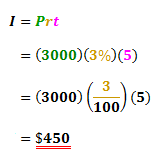# 3010 Simple Interest Summary Quiz

185 Questions | Attempts: 78
ShareSettingsThe simple interest formula is given below in its standard form. When asked for the amount of interest to be paid, this is the perfect formula to use. However, sometimes you will be required to find the principal, rate or time period for a loan, so rearranging this formula may make things simpler. If you are finding the principal, you may rearrange the equation so P is on the left of the equation, and I, r and t are on the right. This is sometimes called 'making P the subject'. You calculator can do this easily for you. This quiz tests your ability to find the Interest, Principal, rate, time and total amount on simple interest problems.

• 1.
I borrow \$17000 and pay interest of \$12240 at 12% per annum. Over what period of time (in years) did I take out the loan? (Just a number is sufficient)
• 2.
I borrow \$19000 and pay interest of \$12540 at 6% per annum. Over what period of time (in years) did I take out the loan? (Just a number is sufficient)
• 3.
I borrow \$93000 and pay interest of \$39060 at 3% per annum. Over what period of time (in years) did I take out the loan? (Just a number is sufficient)
• 4.
I borrow \$77000 and pay interest of \$75460 at 7% per annum. Over what period of time (in years) did I take out the loan? (Just a number is sufficient)
• 5.
I borrow \$24000 and pay interest of \$37440 at 13% per annum. Over what period of time (in years) did I take out the loan? (Just a number is sufficient)
• 6.
I borrow \$95000 and pay interest of \$38000 at 5% per annum. Over what period of time (in years) did I take out the loan? (Just a number is sufficient)
• 7.
I borrow \$6000 and pay interest of \$3840 at 8% per annum. Over what period of time (in years) did I take out the loan? (Just a number is sufficient)
• 8.
I borrow \$16000 and pay interest of \$40800 at 17% per annum. Over what period of time (in years) did I take out the loan? (Just a number is sufficient)
• 9.
I borrow \$94000 and pay interest of \$97760 at 8% per annum. Over what period of time (in years) did I take out the loan? (Just a number is sufficient)
• 10.
I borrow \$8000 and pay interest of \$7040 at 11% per annum. Over what period of time (in years) did I take out the loan? (Just a number is sufficient)
• 11.
I borrow \$21000 and pay interest of \$38220 at 13% per annum. Over what period of time (in years) did I take out the loan? (Just a number is sufficient)
• 12.
I borrow \$22000 and pay interest of \$22000 at 10% per annum. Over what period of time (in years) did I take out the loan? (Just a number is sufficient)
• 13.
I borrow \$42000 and pay interest of \$29400 at 7% per annum. Over what period of time (in years) did I take out the loan? (Just a number is sufficient)
• 14.
I borrow \$65000 and pay interest of \$23400 at 3% per annum. Over what period of time (in years) did I take out the loan? (Just a number is sufficient)
• 15.
I borrow \$64000 and pay interest of \$66560 at 13% per annum. Over what period of time (in years) did I take out the loan? (Just a number is sufficient)
• 16.
I borrow \$54000 and pay interest of \$37800 at 5% per annum. Over what period of time (in years) did I take out the loan? (Just a number is sufficient)
• 17.
I borrow \$41000 and pay interest of \$34440 at 7% per annum. Over what period of time (in years) did I take out the loan? (Just a number is sufficient)
• 18.
I borrow \$50000 and pay interest of \$108000 at 18% per annum. Over what period of time (in years) did I take out the loan? (Just a number is sufficient)
• 19.
I borrow \$31000 and pay interest of \$13640 at 11% per annum. Over what period of time (in years) did I take out the loan? (Just a number is sufficient)
• 20.
I borrow \$48000 and pay interest of \$21120 at 4% per annum. Over what period of time (in years) did I take out the loan? (Just a number is sufficient)
• 21.
I borrow \$46000 and pay interest of \$27600 at 12% per annum. Over what period of time (in years) did I take out the loan? (Just a number is sufficient)
• 22.
I borrow \$88000 and pay interest of \$63360 at 8% per annum. Over what period of time (in years) did I take out the loan? (Just a number is sufficient)
• 23.
I borrow \$69000 and pay interest of \$103500 at 15% per annum. Over what period of time (in years) did I take out the loan? (Just a number is sufficient)
• 24.
I borrow \$85000 and pay interest of \$61200 at 18% per annum. Over what period of time (in years) did I take out the loan? (Just a number is sufficient)
• 25.
I borrow \$52000 and pay interest of \$101400 at 15% per annum. Over what period of time (in years) did I take out the loan? (Just a number is sufficient)

## Related TopicsBack to top
×

Wait!
Here's an interesting quiz for you.